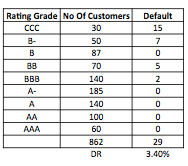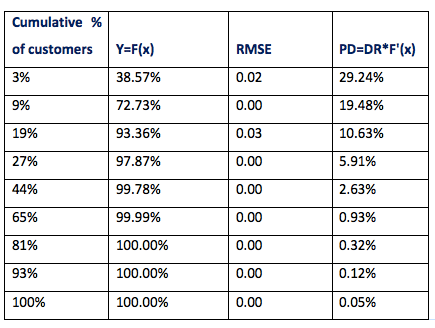top of pageSearch

# Credit Risk Modeling For Low Default Portfolios (LDP)One of the most challenging situations in Credit Risk Modeling is dealing with portfolios that have zero or very low default history.

For modeling the probability of default (PD), banks require robust historical data. In the absence of historical data, it is challenging to estimate the true nature of the portfolio in terms of PD. Also, for any portfolio, the volatility of observed default for many years might be very low or zero but that doesn't mean it will not default in an economic downturn, which implies true PD > 0.

It is also essential for banks to hold enough Capital Reserve (CR) for low default portfolios, at the same time they should not hold too much CR which will cut profit from their books. Hence, it is necessary for the banks to estimate & calibrate the PD for LDP, so they can avoid underestimating/overestimating the CR.

As per Basel, floor PD rate is 0.03% to 0.05%

Zero Default History (Independent):

Consider a portfolio rated Grade A and B with frequencies nA, nB & probability of default pA, pB respectively, which are independent (assumptions).

pA<=pB ..............................(1) Considering the risk of the rating grades.

The most prudent estimate of pB can be obtained by assuming pB=pA, i.e. they are no different in terms of risk and may be considered as homogeneous portfolios with a total size (nA+nB).

The probability of not observing any default at a given confidence level "alpha" cannot be less than (1-alpha). The probability of observing no default can be written as:

(1-pA)^ (nA+nB) >= 1-alpha.............................(2)

from (2) : pA <= 1-(1-alpha)^(1/(nA+nB)) , which is the confidence region for observing default.

Since there is no upper limit for pB, the confidence region for pB can be obtained when it satisfies the below inequality.

(1-pB)^ nB>= 1-alpha ==>> pB <= 1-(1-alpha)^(1/nB)………………..(3)

Apart from the confidence level, the sample size is also a major driver for upper confidence limit. When the sample size increases, it is highly likely the creditworthiness of the portfolio increases but at the same time, we might also observe few default cases.

Low Default History (independent):

As we know, the probability of getting "k" success out of "n" trials follows a Binomial distribution, Hence if we take default events as a "success" and the size of the portfolio as "number of trails" we can use the PMF of the binomial distribution (subject to default event: Independent) as the probability of default estimation.

P(x=k) = nCk*p^k*(1-p)^(n-k) , p= probability of default.

Let's suppose the portfolio is:

A 0 100

B 1 300

C 1 500

D 1 300

Total 3 1200

The probability of observing not more than 3 defaults can be written as :

SUM(i=0 to 3) 1200Ci*p^i*(1-p)^1200-i >= 1-alpha .......)

The following table is prepared by solving the P value at different confidence level (alpha) using Goal seek function in excel.

Table:1

Alpha 50% 75% 90% 95% 99%

P(A) 0.31%. 0.43% 0.56% 0.64% 0.83%

P(B) 0.33% 0.46% 0.61% 0.70% 0.90%

P(C) 0.46% 0.65% 0.83% 0.97% 1.62%

P(D) 0.56% 0.90% 1.29% 1.57% 2.19%

In case, default events are dependent: Pluto proposed Vasicek model with one factor Gaussian correlation parameter (rho)

The conditional probability by Vasicek model is given by :

G(P,rho,y)= Function(y) {dnorm((qnorm(P)-sqrt(rho)*y))/sqrt(1-rho))} ........ (5)

dnorm (in R) =NORM.DIST

qnorm(in R)=NORM.INV

Where rho=0.12 minimum as per Basel

Now using the conditional probability from equation (5) as P in the Binomial distribution we get the following conditional PMF:

P(x=k,Y=y) = nCk*G(p,rho,y)^k*(1-G(p,rho,y))^(n-k) .............(6)

In order to make equation (6) as the unconditional probability of default, y has to be removed and it will be transformed into the following PMF :

INT<-function(y){dnorm(y)*nCk*G(p,rho,y)^k*(1-G(p,rho,y))^(n-k)

integrate(INT, lower =-Inf, upper = Inf)}......................(7)

Finally, The equation (7) is used to calculate the probability of default for different grades considering the respective default events for each existing grades & rho=0.12. The results after solving the equation are given below:

Table 2:

alpha. 50%. 75%. 90%. 95% 99%

P(A) 0.50% 1.02% 1.81% 2.56% 4.43%

P(B) 0.54% 1.10% 1.94% 2.73% 4.70%

P(C) 0.54% 1.12% 2.00% 2.83% 4.92%

P(D) 0.84% 1.77% 3.17% 4.45% 7.64%

The above calculation can easily be done with R

Comparing Table 1 & 2, demonstrates the visible effect of asset correlation on each rating grades within the portfolio while estimating the probability of default.

Cumulative Accuracy Profile (CAP)

Another approach of calculating PD for a low default portfolio is using the CAP curve of an existing rating model. CAP curve is also known as Gini Chart or Power Curve in marketing analytics and other industries. It helps the model developer to judge the discriminatory power of their models. By using CAP performance, current model are compared against perfect & random models. The Area under the current model and random models are compared with area under the perfect model and random model, which is also know an Accuracy ratio (AR).

Deriving the CAP curve:

Let’s suppose we have the following rating class with respective customers and default numbers in our portfolio. In order to derive the CAP curve, these data points are arranged in descending order of risk.

Actual modelThe CAP curve of the rating model will be in between a Perfect model & Random model, which can be derived from the table below.CAP curve:The mathematical expression of CAP curve can be written as:

Y= [1-e^(-k*x)]/(1-e^(-k))………………………………1

Where y is the cumulative % of bad customers from top to bottom.

Where x is the cumulative % of customers from top to bottom.

Where k is the concavity. Higher k shows good discriminatory power of the model i.e. more concave in nature.

The PD can be derived by using the following equation of Falkenstein et al

PD(r)=D*dy/dx , r is the rating class……………………….2

dy/dx= rate of change of default with change in borrowers number in a rating class

(1)&(2) gives:

PD(r)= DR*(k*e^(-k*x)/(1-e^-k)…………………………3

Before deriving the PD , we need to calculate concavity (k). Here K is derived by minimizing the RMSE of estimated CAP by using the equation(1) and observed CAP (cumulate % of bad customers) using Solver.

DR is nothing but the average of observed default customers to the whole portfolio.(3.4% refer to first table)

Now by using the equation 3 the following PD of respective rating class can be derived.The derived PD for each rating class is shown in the chart below.Article Author: Ramen Borah, Business Analyst, Care Risk Solutions.